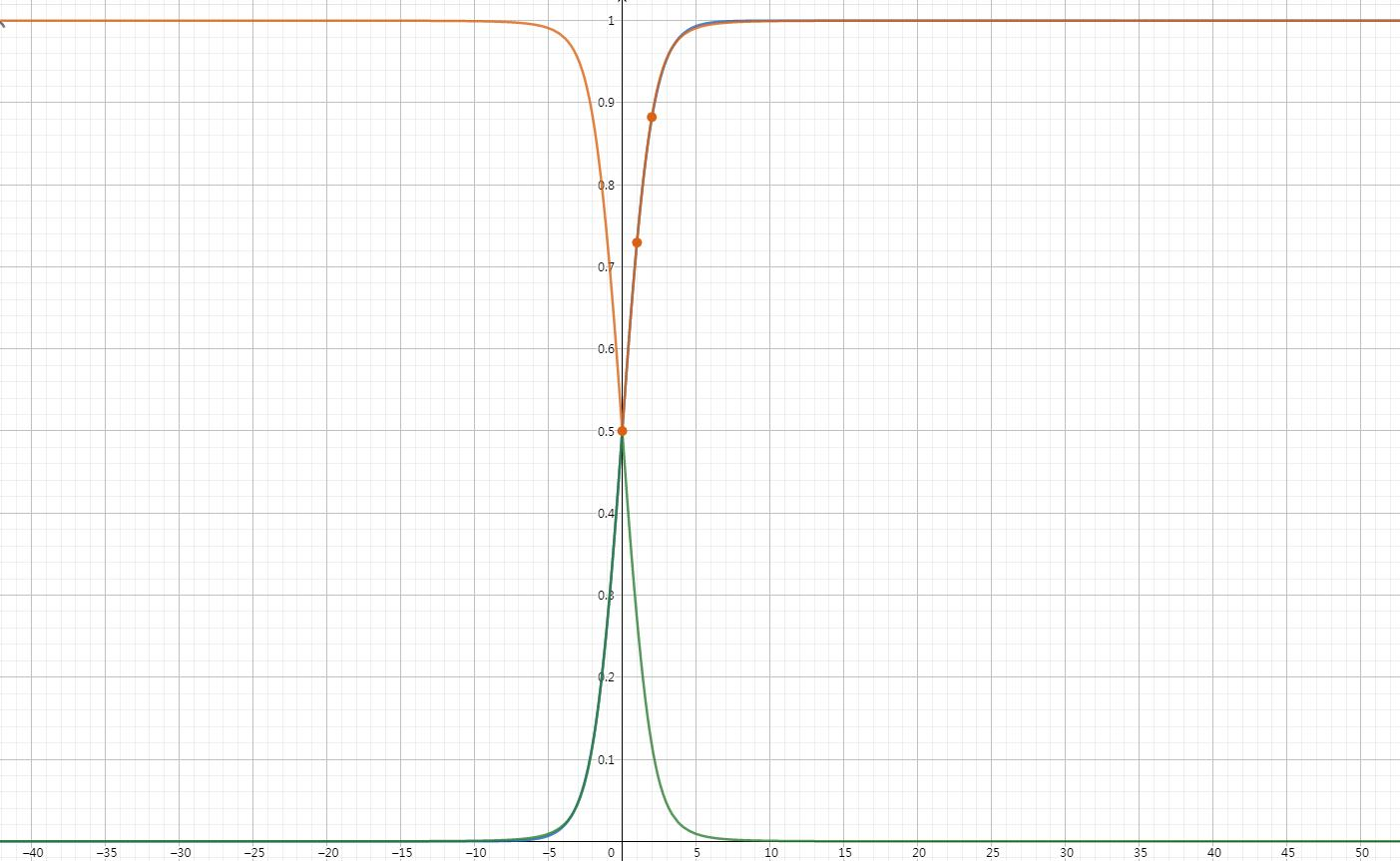# 1 Sigmoid

Sigmoid激活函数的公式：

sigmoid(x) = \frac{1}{1+e^{-x} }

sigmoid(x) = \frac{1}{1+e^{-x} } = \frac{e^{x} }{1+e^{x}} = \frac{1}{2} tanh(\frac{x}{2} )+\frac{1}{2}

Sigmoid激活函数的函数曲线：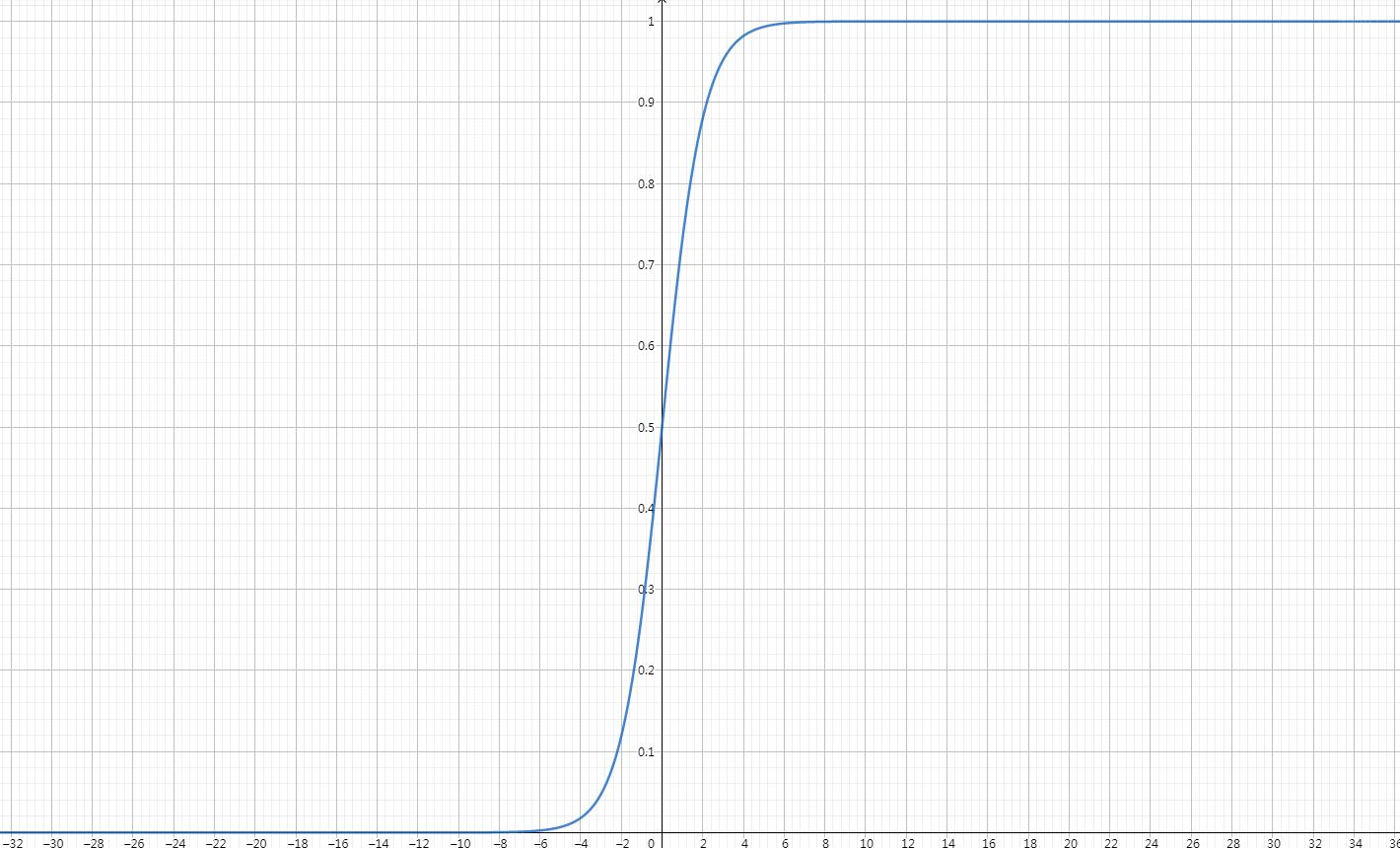float sigmoid(float x)
{
return (1 / (1 + exp(-x)));
}


# 2 Sigmoid的近似与快速替代方案

## 2.1 方案1

sigmoid-replace(x) = \frac{1}{2} (\frac{x}{1+\left | x \right | } )+\frac{1}{2}

float sigmoid(float x)
{
return 0.5 * (x / (1 + fabs(x))) + 0.5;
}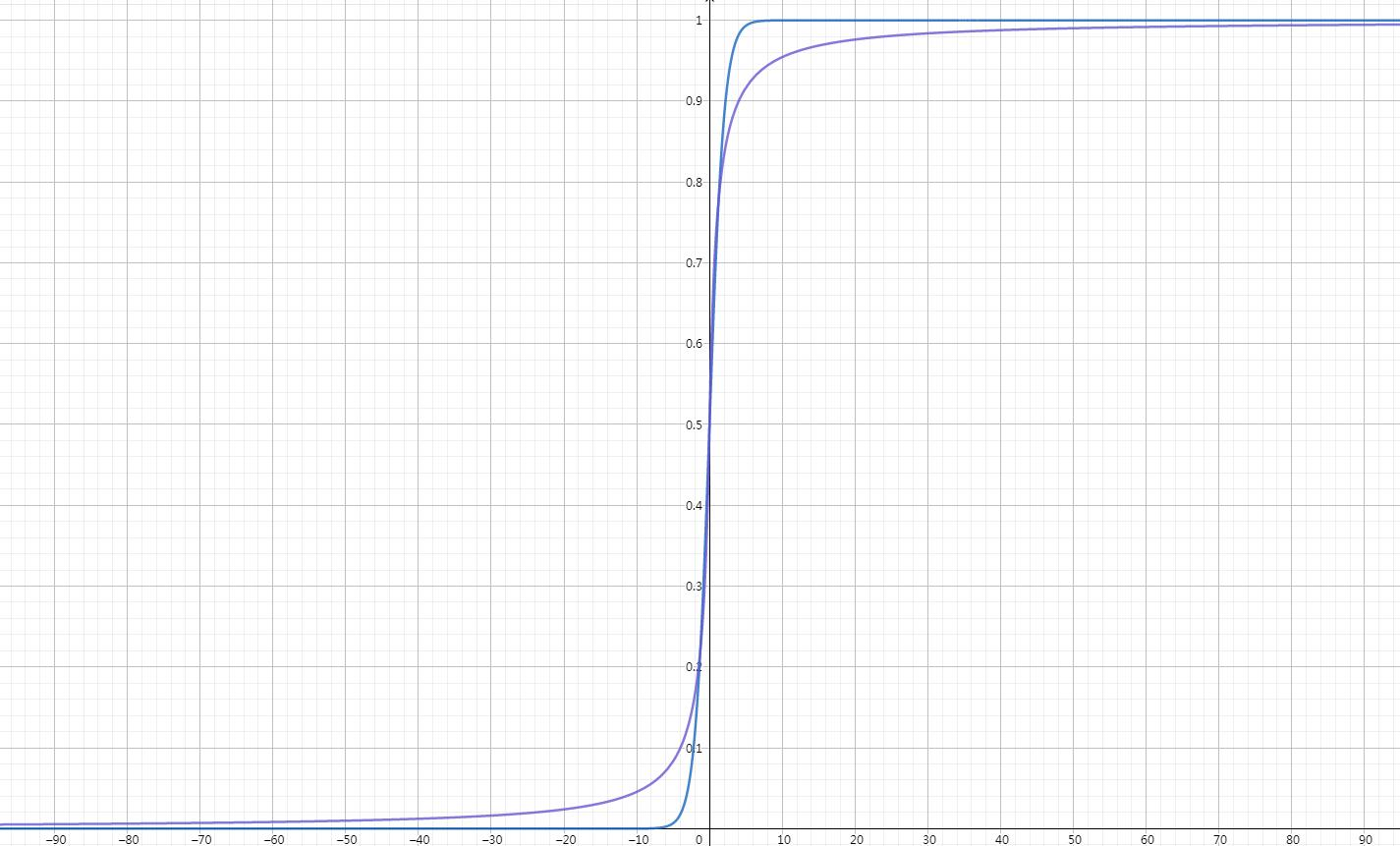## 2.2 方案2

sigmoid-replace(x) = \frac{1}{2} (\frac{x}{\frac{0.99}{\left | x \right | } +\left | x \right | } )+\frac{1}{2}

float sigmoid(float x)
{
return 0.5*(x/(0.99/abs(x) + abs(x))) + 0.5;
}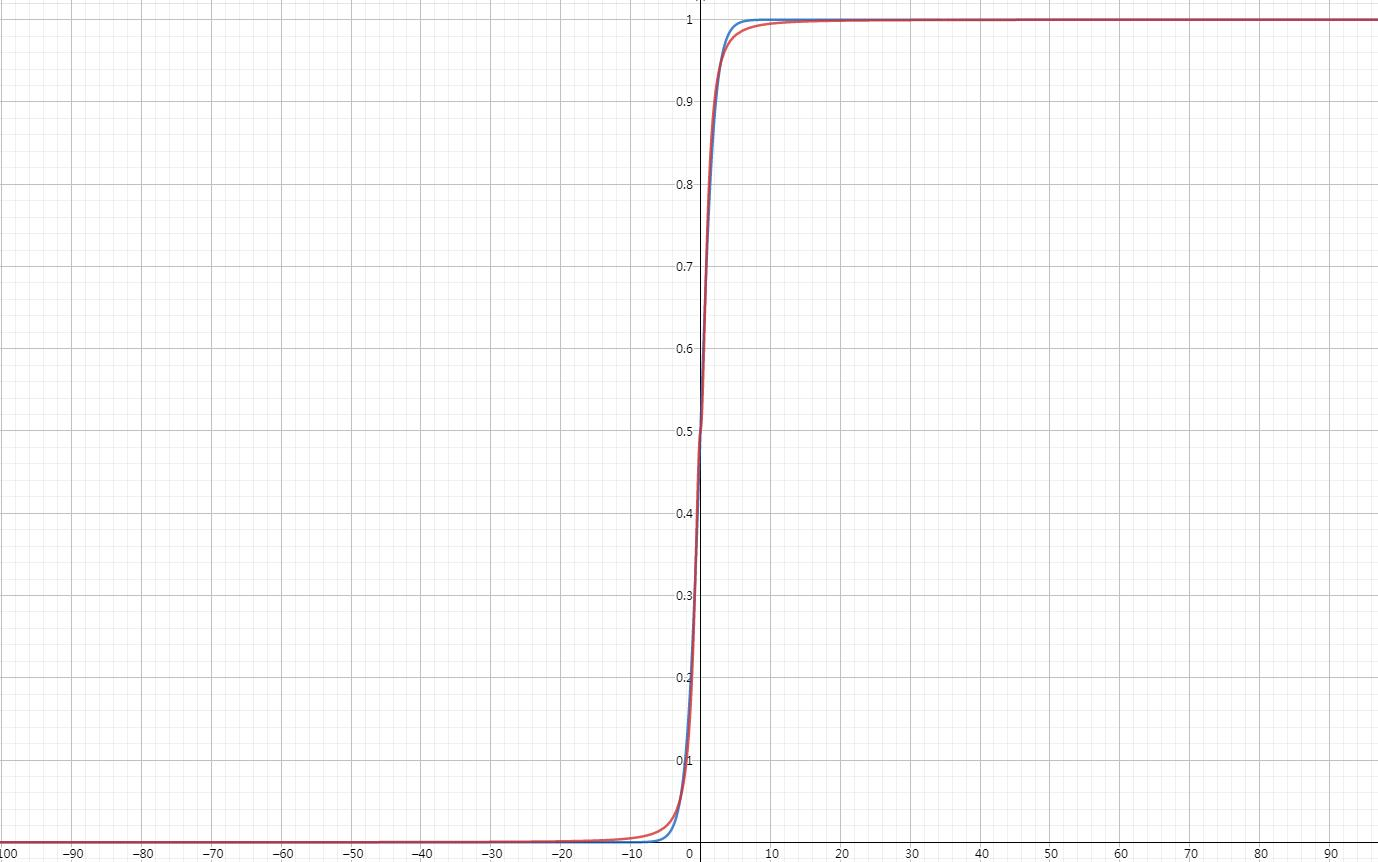\frac{0.99}{\left | x \right | }

## 2.3 方案3

sigmoid-replace(x) = \frac{1}{2} tanh(\frac{x}{2} )+\frac{1}{2}

float sigmoid(float x)
{
return 0.5 + 0.5 * tanh(x / 2);
}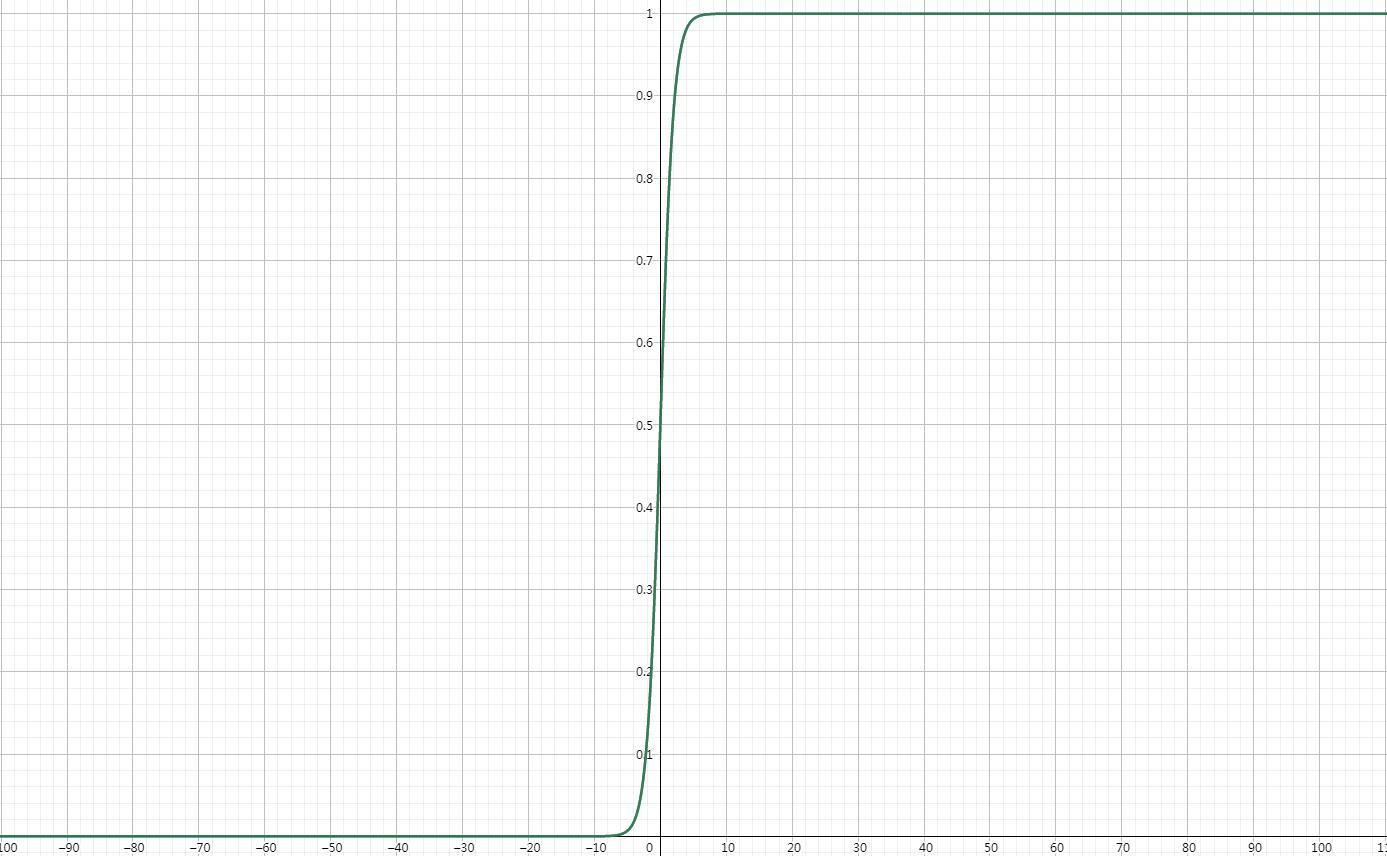## 2.4 方案4

\begin{array}{c}
\left\{\begin{matrix}
\frac{1}{1+\frac{1}{1+\left | x \right | +x^{2}\times 0.555+x^{4}\times 0.143 } } & x \ge 0\\
\frac{1}{1+{1+\left | x \right | +x^{2}\times 0.555+x^{4}\times 0.143 }} & x < 0
\end{matrix}\right.
\end{array}